# Samacheer Kalvi Books: Tamilnadu State Board Text Books Solutions

## Samacheer Kalvi 10th Science Chapter 2 Optics Notes PDF Download: Tamil Nadu STD 10th Science Chapter 2 Optics NotesSamacheer Kalvi 10th Science Chapter 2 Optics Notes PDF Download: Tamil Nadu STD 10th Science Chapter 2 Optics Notes

We bring to you specially curated Samacheer Kalvi 10th Science Chapter 2 Optics Notes PDF which have been prepared by our subject experts after carefully following the trend of the exam in the last few years. The notes will not only serve for revision purposes, but also will have several cuts and easy methods to go about a difficult problem.

 Board Tamilnadu Board Study Material Notes Class Samacheer Kalvi 10th Science Subject 10th Science Chapter Chapter 2 Optics Format PDF Provider Samacheer Kalvi Books

## How to Download Samacheer Kalvi 10th Science Chapter 2 Optics Notes PDFs?

2. Click on the Samacheer Kalvi 10th Science Notes PDF.
3. Look for your preferred subject.
4. Now download the Samacheer Kalvi 10th Science Chapter 2 Optics notes PDF.

## Download Samacheer Kalvi 10th Science Chapter 2 Optics Chapterwise Notes PDF

### Samacheer Kalvi 10th Science Optics Textual Solved Problems

10th Science Optics Book Back Answers Question 1.
Light rays travel from vacuum into a glass whose refractive index is 1.5. If the angle of incidence is 30°, calculate the angle of refraction inside the glass.
Solution:
According to Snell’s law,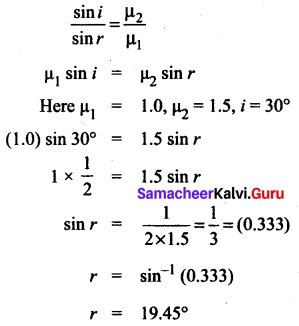Optics Lesson Book Back Answers Question 2.
A beam of light passing through a diverging lens of focal length 0.3 m appears to be focused at a distance 0.2 m behind the lens. Find the position of the object.
Solution:
f = -0.3 m, v = -0.2 m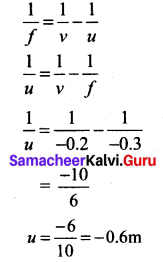10th Science Optics Lesson Question 3.
A person with myopia can see objects placed at a distance of 4 m. If he wants to see objects at a distance of 20 m, what should be the focal length and power of the concave lens he must wear?
Solution:
Given that x = 4 m and y = 20 m.
Focal length of the correction lens is, f=xyx−y
f=4×204−20=80−16=−5m
Power of the correction lens =1f=−15=−0.2D.

Optics 10th Class Question 4.
For a person with hypermetropia, the near point has moved to 1.5 m. Calculate the focal length of the correction lens in order to make his eyes normal.
Solution:
Given that, d = 1.5 m; D = 25 cm = 0.25 m (For a normal eye).
The focal length of the correction lens is,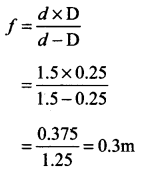### Samacheer Kalvi 10th Science Optics Textual Evaluation

10th Standard Optics Lesson Question 1.
The refractive index of four substances A, B, C and D are 1.31, 1.43, 1.33, 2.4 respectively. The speed of light is maximum in ____.
(a) A
(b) B
(c) C
(d) D.
(a) A

10th Optics Lesson Question 2.
A small bulb is placed at the principal focus of a convex lens. When the bulb is switched on, the lens will produce:
(a) a convergent beam of light
(b) a divergent beam of light
(c) a parallel beam of light
(d) a coloured beam of light
(a) a convergent beam of light

Class 10 Science Optics Question 3.
A small bulb is placed at the principal focus of a convex lens. When the bulb is switched on, the lens will produce ____.
(a) a convergent beam of light
(b) a divergent beam of light
(c) a parallel beam of light
(d) a coloured beam of light.
(c) a parallel beam of light

Samacheer Kalvi Guru 10th Science Question 4.
Magnification of a convex lens is:
(a) Positive
(b) negative
(c) either positive or negative
(d) zero
(a) Positive

Samacheer Kalvi 10th Science Solutions Question 5.
A convex lens forms a real, diminished point sized image at the focus. Then the position of the object is at _____.
(a) focus
(b) infinity
(c) at 2f
(d) between f and 2f.
(b) infinity

Samacheer Kalvi 10th Science Solution Question 6.
Power of a lens is -4D, then its focal length is _____.
(a) 4m
(b) -40m
(c) -0.25 m
(d) -2.5 m.
(c) -0.25m

10th Science Samacheer Kalvi Question 7.
In a myopic eye, the image of the object is formed:
(a) behind the retina
(b) on the retina
(c) in front of the retina
(d) on the blind spot
(c) in front of the retina

Science Solution Class 10 Samacheer Kalvi Question 8.
The eye defect ‘presbyopia’ can be corrected by ______.
(a) convex lens
(b) concave lens
(c) convex mirror
(d) bifocal lenses.
(d) bifocal lenses.

Samacheer Kalvi 10th Science Question 9.
Which of the following lens would you prefer to use while reading small letters found in a dictionary?
(a) A convex lens of focal length 5 cm
(b) A concave lens of focal length 5 cm
(c) A convex lens of focal length 10 cm
(d) A concave lens of focal length 10 cm.
(c) A convex lens of focal length 10 cm

10th Science Solution Samacheer Kalvi Question 10.
If VB, VG, VR be the velocity of blue, green and red light respectively in a glass prism, then which of the following statement gives the correct relation?
(a) VB = VG = VR
(b) VB > VG > VR
(c) VB < VG < VR
(d) VB < VG > VR
(c) VB < VG < VR

II. Fill in the Blanks.

Samacheer Kalvi Guru 10th Science Book Back Answers Question 1.
The path of the light is called as ______.
ray of light.

Samacheer Kalvi 10 Science Question 2.
The refractive index of a transparent medium is always greater than _____.
one.

10th Samacheer Kalvi Science Question 3.
If the energy of the incident beam and the scattered beam are the same, then the scattering of light is called _____ scattering.
elastic.

Samacheer Kalvi 10th Science Solution Book Question 4.
According to Rayleigh’s scattering law, the amount of scattering of light is inversely proportional to the fourth power of its ____.
wavelength.

Question 5.
Amount of light entering into the eye is controlled by ______.
Iris.

III. True or False. If False Correct it.

Question 1.
The velocity of light is greater in a denser medium than in a rarer medium?
False.
Correct Statement: Velocity of light is lesser in a denser medium than in rarer medium

Question 2.
The power of the lens depends on the focal length of the lens?
True.

Question 3.
Increase in the converging power of eye lens cause ‘hypermetropia’.
True.

Question 4.
The convex lens always gives a small virtual image.
False.
Correct Statement: The convex lens give enlarged. Virtual image when it is placed between pole and principal focus.

IV. Match the Following.

Question 1.

 1. Retina (a) Pathway of light 2. Pupil (b) Far point comes closer 3. Ciliary muscles (c) Near point moves away 4. Myopia (d) Screen of the eye 5. Hypermetropia (e) Power of accommodation

1. (d) Screen of the eye
2. (a) Pathway of light
3. (e) Power of accommodation
4. (b) Far point comes closer
5. (c) Near point moves away

V. Assertion and Reasoning Type Questions

Mark the correct choice as?
(a) If both assertion and reason are true and reason is the correct explanation of assertion.
(b) If both assertion and reason are true but reason is not the correct explanation of assertion.
(c) Assertion is true but reason is false.
(d) Assertion is false but the reason is true.

Question 1.
Assertion: If the refractive index of the medium is high (denser medium) the velocity of the light in that medium will be small
Reason: Refractive index of the medium is inversely proportional to the velocity of the light
(a) If both assertion and reason are true and reason is the correct explanation of assertion.

Question 2.
Assertion: Myopia is due to the increase in the converging power of the eye lens.
Reason: Myopia can be corrected with the help of the concave lens.
(b) If both assertion and reason are true but reason is not the correct explanation of assertion.

Question 1.
What is refractive index?
The ratio of the speed of light in a vacuum to the speed of light in a medium is defined as the refractive index ‘µ’ of that medium.

Question 2.
State Snell’s law.
The ratio of the sine of the angle of incidence and sine of the angle of refraction is equal to the ratio of refractive indices of the two media. This law is also known as Snell’s law.
sinisinr=μ2μ1

Question 3.
Draw a ray diagram to show the image formed by a convex lens when the object is placed between F and 2F.
Ray diagram for an object placed between F and 2F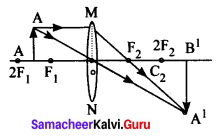Question 4.
Define dispersion of light.
When a beam of white light or composite light is refracted through any transparent media such as glass or water, it is split into its component colours. This phenomenon is called as ‘dispersion of light’.

Question 5.
State Rayleigh’s law of scattering.
Rayleigh’s scattering law states that “The amount of scattering of light is inversely proportional to the fourth power of its wavelength”.
Amount of scattering s∝1λ4

Question 6.
Differentiate convex lens and concave lens.

 Convex Lens Concave Lens 1. The lens which is thicker at the centre than at the edges. 1. The lens is thinner at the centre than at the edges. 2. Abeam of light passing through it, is converged to a point. 2. A beam of light passing through it is diverged or spread out. 3. It is called a converging lens. 3. It is called a diverging lens.

Question 7.
What is power of accommodation of eye?
The ability of the eye lens to focus nearby as well as the distant objects is called power of accommodation of the eye.

Question 8.
What are the causes of ‘Myopia’?
Myopia, also known as short-sightedness, occurs due to the lengthening of the eyeball. With this defect, nearby objects can be seen clearly but distant objects cannot be seen clearly.

Question 9.
Why does the sky appear in blue colour?
When sunlight passes through the atmosphere, the blue colour (shorter wavelength) is scattered to a greater extent than the red colour (longer wavelength). This scattering causes the sky to appear in blue colour.

Question 10.
Why are traffic signals red in colour?

1. The wavelength of red colour is more than other colours.
2. Red colour will travel longer distance without scattering.
3. Red colour gets least scattered and reaches people.

VII. Give the Answer in Detail.

Question 1.
List any five properties of light.

1. Light is a form of energy.
2. Light always travels along a straight line.
3. Light does not need any medium for its propagation. It can even travel through vacuum.
4. The speed of light in vacuum or air is, c = 3 × 108 ms-1.
5. Since, light is in the form of waves, it is characterized by a wavelength (k) and a frequency (v), which are related by the following equation: c = v λ (c – velocity of light).
6. Different coloured light has different wavelength and frequency.
7. Among the visible light, violet light has the lowest wavelength and red light has the highest wavelength.
8. When light is incident on the interface between two media, it is partly reflected and partly refracted.

Question 2.
Explain the rules for obtaining images formed In a convex lens with the help of a ray diagram.
Rule 1: When a ray of light strikes the convex or concave lens obliquely at its optical centre, it continues to follow its path without any deviation.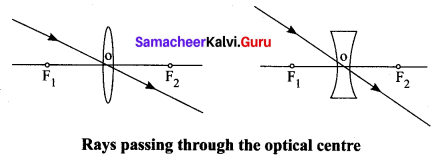Rule 2: When rays parallel to the principal axis strikes a convex or concave lens, the refracted rays are converged to (convex lens) or appear to diverge from (concave lens) the principal focus.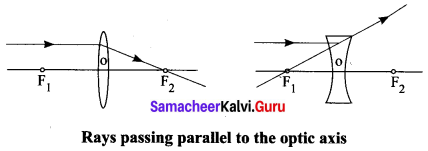Rule 3: When a ray passing through (convex lens) or directed towards (concave lens) the principal focus strikes a convex or concave lens, the refracted ray will be parallel to the principal axis.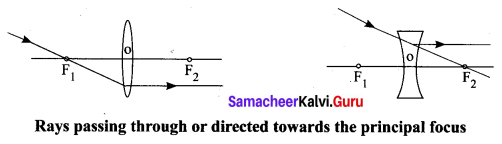Question 3.
Differentiate the eye defects: Myopia and Hypermetropia?

 Myopia Hypermetropia (i) Myopia, also known as short-sightedness, occurs due to the lengthening of the eyeball. (i) Hypermetropia, also known as long-sightedness, occurs due to the shortening of the eyeball. (ii) With this defect, nearby objects can be seen clearly but distant objects cannot be seen clearly. (ii) With this defect, distant objects can be seen clearly but nearby objects cannot be seen clearly. (iii) The focal length of the eye lens is reduced or the distance between the eye lens and retina increases. (iv) The focal length of the eye lens is increased or the distance between the eye lens and retina decreases. (iv) The far point will not be infinity for such eyes and the far point has come closer. (iv) Hence, the near point will not be at 25 cm for such eyes and the near point has moved farther. (v) Due to this, the image of distant objects is formed before the retina. (v) Due to this, the image of nearby objects is formed behind the retina. (vi) This defect can be corrected using a concave lens (vi) This defect can be corrected using a convex lens.

Question 4.
Explain the construction and working of a ‘Compound Microscope’.
Construction:

• A compound microscope consists of two convex lenses. The lens with the shorter focal length is placed near the object, and is called an ‘objective lens’ or ‘objective piece’.
• The lens with larger focal length and larger aperture placed near the ‘observer’s eye is called as ‘eye lens’ or ‘eyepiece’.
• Both the lenses are fixed in a narrow tube with adjustable provision.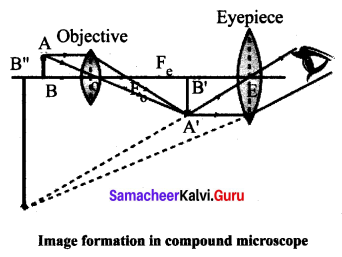Working:

• The object (AB) is placed at a distance slightly greater than the focal length of the objective lens (u > f0).
• A real, inverted and magnified image (A’B’) is formed at the other side of the objective lens.
• This image behaves like the object for the eye lens. The position of the eye lens is adjusted in such a way, that the image (A’B’) falls within the principal focus of the eyepiece.
• This eyepiece forms a virtual, enlarged and erect image (A” B”) on the same side of the object.
• A compound microscope has 50 to 200 times more magnification power than the simple microscope.

VIII. Numerical Problems:

Question 1.
An object is placed at a distance 20 cm from a convex lens of focal length 10 cm. Find the image distance and nature of the image.
Solution: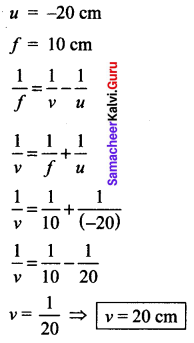Nature of the image real enlarged and inverted image.

Question 2.
An object of height 3 cm is placed at 10 cm from a concave lens of focal length 15 cm. Find the size of the image.
Solution:
u = -10 cm [left side of lens]
f = -15 cm [left side of lens]
h = 3 cm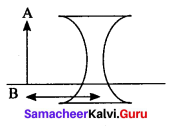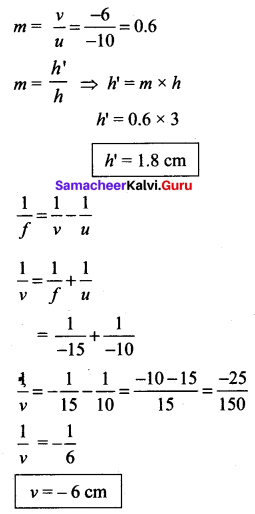Nature of image: When an object is placed at 10 cm from the left side of the lens, a virtual image is formed between the optical centre and focus of a concave lens. The size of the image is smaller than that of the object.

IX. Higher-Order Thinking (HOT) Questions:

Question 1.
While doing an experiment for the determination of the focal length of a convex lens, Raja Suddenly dropped the lens. It got broken into two halves along the axis. If he continues his experiment with the same lens,

1. Can he get the image?
2. Is there any change in the focal length?

1. Yes, he can get the image, because the lens is broken into two halves along the axis.
2. No, focal length remains the same for the lens if it’s broken (or) not broken.

Question 2.
The eyes of the nocturnal birds like owl are having a large cornea and a large pupil. How does it help them?

1. Owl has large pupil so that it provides a larger pathway for light to flow towards the retina.
2. In addition it has large comer so that a bigger image of the insects would be formed on the retina.
3. In this way the eyes of the nocturnal birds help them to identify the elements in the surroundings.

### Samacheer Kalvi 10th Science Optics Additional Questions

Question 1.
The speed of light in vacuum or air is _____.
(a) 3 × 10-8 ms-1
(b) 3 × 108 ms-1
(c) 3 × 108 m-1s
(d) 3 × 10-18 ms-1.
(b) 3 × 108 ms-1

Question 2.
If the energy of the incident beam of light and the scattered beam of light are same, then it is known as:
(a) Inelastic scattering
(b) Elastic scattering
(c) Raman scattering
(d) Mie scattering
(b) Elastic scattering

Question 3.
Which is the following is not an example of colloid _____.
(a) milk
(b) ice – cream
(c) pure water
(d) smoke.
(c) pure water

Question 4.
The band of colours is termed as _____.
(a) monochromatic source
(b) composite light
(c) spectrum
(d) dispersion of light.
(c) spectrum

Question 5.
Water droplets, pollen and dust cause scattering.
(a) Elastic
(b) Inelastic
(c) Mie
(d) Rayleigh
(c) Mie

Question 6.
_____ scattering takes place when the diameter of the Scatterer is similar to or larger than the wavelength of the incident light.
(a) Rayleigh
(b) Tyndall
(c) Mie
(d) Raman.
(c) Mie

Question 7.
The colour of the sun is red at sunrise and sunset. This occurs due to _____ scattering.
(a) Rayleigh
(b) Mie
(c) Tyndall
(d) Raman.
(a) Rayleigh

Question 8.
A lens may be considered to be made up of:
(a) lenses
(b) prisms
(c) mirrors
(d) transparent medium
(b) prisms

Question 9.
If one of the faces of a bi-convex lens is plane, it is known as a ______.
(a) convex lens
(b) Plano-convex lens
(c) concave lens
(d) Plano concave lens.
(b) Plano-convex lens

Question 10.
The spectral lines having a frequency equal to the incident ray frequency is called ______.
(a) Spectral lines
(b) Raman lines
(c) Colour lines
(d) Rayleigh lines.
(d) Rayleigh lines.

Question 11.
The lens that is thinner in the middle than at the edges is called:
(a) concave lens
(b) bifocal lens
(c) cylindrical lens
(d) convex lens
(a) concave lens

Question 12.
Magnification of a concave lens is ______.
(a) positive
(b) negative
(c) either positive or negative
(d) zero.
(b) negative

Question 13.
Where should an object be placed so that a real image is formed at infinity is obtained by a convex lens _____.
(a) 2F
(b) 0
(c) F
(d) >2F.
(c) F

Question 14.
The focal length of a lens is the distance between:
(a) optic centre and principal focus
(b) optic centre and centre of curvature
(c) principal focus and centre of curvature
(d) none
(a) optic centre and principal focus

Question 15.
Lens Maker’s formula is _____.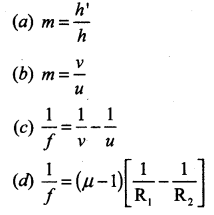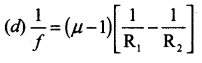Question 16.
The power of a lens is numerically defined as the reciprocal of its _____.
(a) wavelength
(b) frequency
(c) focal length
(d) refractive index.
(c) focal length

Question 17.
When the object is placed at the principal focus of concave lens, the image will be formed at:
(a) 2F
(b) F
(c) infinity
(d) between F and 2F
(c) infinity

Question 18.
Astigmatism can be corrected by using _____ lens.
(a) convex
(b) concave
(c) cylindrical
(d) bifocal.
(c) cylindrical

Question 19.
The least distance of distinct vision for the normal human eye is ______.
(a) 30 cm
(b) 25 cm
(c) 35 cm
(d) infinity.
(b) 25 cm

Question 20
To get a real and diminished image using convex lens, the object must be placed:
(a) at F
(b) beyond 2F
(c) at 2F
(d) beyond F
(b) beyond 2F

II. Fill in the Blanks.

Question 1.
The interacting particle of the medium is called ______.
Scatterer.

Question 2.
When a beam of light is refracted through any transparent media, it split into its component colours. This is called as _____.
dispersion of light.

Question 3.
The speed of light in a medium is high if the refractive index of the medium is _____?
low.

Question 4.
The spectral lines which are having frequencies other than the incident ray frequency are called _____?
Raman lines.

Question 5.
_____ lenses are used to correct the defect of vision called hypermetropia.
Convex.

Question 6.
______ lenses are used as the eye lens of the Galilean Telescope.
Concave.

Question 7.
By convention, the power of a convex lens is taken as _____ whereas the power of a concave lens is taken as _____
positive, negative.

Question 8.
The diameter of a human eye is _____?
2.3 cm.

Question 9.
_____ is achieved by changing the focal length of the eye lens with the help of ciliary muscles.
Power of accommodation.

Question 10.
_____ is the coloured part of an eye.
Iris.

Question 11.
______ is used to observe parts of flower, insects and fingerprints in the field of forensic science.
Simple microscope.

Question 12.
A _____ works based on the principle of the vernier.
travelling microscope.

Question 13.
A _____ telescope is used to view heavenly bodies like stars, planets galaxies and satellites.
astronomical.

Question 14.
_______ is an optical instrument used to see distant objects clearly.
Telescope.

Question 15.
_______ is the most sensitive part of the human eye.
Retina.

Question 16.
A convex lens is ______ in the middle than at edges.
Thicker.

Question 17.
_____ lens produces mostly real images.
Convex.

Question 18.
The SI unit of power of a lens is _____.
dioptre.

Question 19.
The amount of scattering of light is _____ proportional to the fourth power of its wavelength.
inversely.

Question 20.
Refractive index of the medium is dependent on the ______ of the light.
wavelength.

III. True or False. If False Correct it.

Question 1.
Light always travels along a straight line.
True.

Question 2.
The incident ray, the refracted ray of light and the normal to the refracting surface all lie in the different plane.
False.
Correct Statement: The incident ray, the refracted ray of light and the normal to the refracting surface all lie in the same plane.

Question 3.
The angle of refraction is the same for different colours.
False.
Correct Statement: The angle of refraction is different for different colours.

Question 4.
The scattering of light rays by the colloidal particles in the colloidal solution is called the Tyndall effect.
True.

Question 5.
The convex lens is also called a diverging lens.
False.
Correct Statement: Concave lens is also called the diverging lens.

Question 6.
Convex lenses are used in making microscope, telescope and slide projectors.
True.

Question 7.
The distances measured against the direction of incident light are taken as negative.
True.

Question 8.
Converging lenses are used in wide-angle spy hole indoors.
False.
Correct Statement: Diverging lenses or concave lenses are used in wide-angle spy hole indoors.

Question 9.
If the magnification is less than 1, then we get a diminished image.
True.

Question 10.
A compound microscope has 50 to 200 times more magnification power than the simple microscope.
True.

IV. Match the following.

Question 1.

 1. Snell’s law (a) 25 cm 2. Rayleigh scattering (b) Diverging lens 3. Mie scattering (c) converging lens 4. Bi-Convex lens (d) Front surface of an eyeball 5. Bi-concave lens (f) Infinity 6. Cornea (g) The sky to appear in blue colour 7. Near point of eye (h) sinisinr=μ2μ1 8. Far point of the eye (i) White appearance of the clouds 9. Presbyopia (j) Torrid lenses 10. Astigmatism (k) Bifocal lenses

1. (h) sinisinr=μ2μ1
2. (g) The sky to appear in blue colour
3. (i) White appearance of the clouds
4. (c) converging lens
5. (b) Diverging lens
6. (d) Front surface of an eyeball
7. (a) 25 cm
8. (f) Infinity
9. (k) Bifocal lenses
10. (j) Torrid lenses

V. Assertion and Reasoning Type Questions

Mark the correct choice as?
(a) If both assertion and reason are true and the reason is the correct explanation of assertion.
(b) If both assertion and reason are true but the reason is not the correct explanation of assertion.
(c) Assertion is true but the reason is false.
(d) Assertion is false but the reason is true.

Question 1.
Assertion: The refractive index of the medium is different for different coloured lights.
Reason: The refractive index of a medium is dependent on the wavelength of the light.
(a) If both assertion and reason are true and reason is the correct explanation of assertion.

Question 2.
Assertion: A parallel beam of light passing through the concave lens, is diverged or spread out.
Reason: A convex lens is also called a diverging lens.
(c) Assertion is true but the reason is false.

Question 3.
Assertion: Magnification power of microscopes can be decreased by increasing the focal length of the lens used.
Reason: Due to constructional limitations, the focal length of the lens cannot be decreased beyond certain unit.
(d) The assertion is false but the reason is true.

Question 1.
Why does the cloud appear in white colour?

• Mie scattering is responsible for the white appearance of the clouds.
• When white light falls on the water drop, all the colours are equally scattered which together form the white light.

Question 2.
Define Raman Scattering.
Raman Scattering is defined as “The interaction of light ray with the particles of pure liquids or transparent solids, which leads to a change in wavelength or frequency.”

Question 3.
Differentiate stokes line and anti-stokes lines.

1. Stokes lines: The lines having frequencies lower than the incident frequency is called stokes lines.
2. Antistokes lines: The lines having frequencies higher than the incident frequency are called Antistokes lines.

Question 4.
What is meant by colloid?
A colloid is a microscopically small substance that is equally dispersed throughout another material.
Example: Milk, Ice cream, muddy water, smoke.

Question 5.
What are the applications of the convex lens?

1. Convex lenses are used as camera lenses.
2. They are used as magnifying lenses.
3. They are used in making microscope, telescope and slide projectors.
4. They are used to correct the defect of vision called hypermetropia.

Question 6.
Give the lens formula.
The lens formula gives the relationship among distance of the object (u), the distance of the image (v) and the focal length (f) of the lens. It is expressed as
1f=1v−1u
It is applicable to both convex and concave lenses.

Question 7.
What are the applications of a concave lens?

1. Concave lenses are used as eye lens of ‘Galilean Telescope’
2. They are used in wide-angle spy hole indoors.
3. They are being used to correct the defect of vision called ‘myopia’

Question 8.
What are the uses of a simple microscope?
Simple microscopes are used

1. By watch repairers and jewellers.
2. To read small letters clearly.
3. To observe parts of flower, insects etc.
4. To observe Anger prints in the field of forensic science.

Question 9.
Define power of a lens.

1. The ability of a lens to converge (convex lens) or diverge (concave lens) is called its power.
2. The power of a lens can be defined as the deep of convergence or divergence of light rays.
3. Power of a lens is numerically defined as the reciprocal of its focal length.
P=1f
The SI unit of power of a lens is dioptre.

Question 10.
Define magnification of a lens.
It is defined as the ratio of the height of the image to the height of an object. Magnification is denoted by the letter ‘m’.If the height of the object is ‘h’ and the height of the image is h’, the magnification produced by a lens is,
m= height of the image  height of the object =h′h
Also, it is related to the distance of the object (w) and the distance of the image (v) as follows:
m= distance of the image  distance of the object =vu

Question 11.
Give the lens maker’s formula.
The lens maker’s formula is one such equation. It is given as
1f=(μ−1)(1R1−1R2)
where µ is the refractive index of the material of the lens; R1 and R2 are the radii of curvature of the two faces of the lens; f is the focal length of the lens.

Question 12.
Based on the initial and final energy of the light beam, classify the scattering.
Based on the initial and final energy of the light beam, scattering can be classified as

• Elastic scattering
• Inelastic scattering

Question 13.
Based on the nature and size of the scatter. Classify the scattering.
The nature and size of the scatterer result in different types of scattering. They are

• Rayleigh scattering
• Mie scattering
• Tyndall scattering
• Raman scattering

Question 14.
Why does the sky appear in red colour at sunrise and sunset?

1. At sunrise and sunset, the light rays from the Sun have to travel a larger distance in the atmosphere than at noon.
2. Most of the blue lights are scattered away and only the red light which gets least scattered reaches us. Therefore, the colour of the Sun is red at sunrise and sunset.

Question 15.

• Elaborate view of the Galaxies, Planets, stars and other heavenly bodies is possible.
• The camera can be attached for taking photographs for the celestial objects.
• The telescope can be viewed even with the low intensity of light.

• Frequent maintenances needed.
• It is not easily portable one.

VII. Give the Answer in Detail.

Question 1.
What is scattering? Explain two types of scattering.
When a beam of light, interacts with a constituent particle of the medium, it undergoes many kinds of scattering. Based on initial and final energy of the light beam,scattering can be classified as,
(i) Elastic scattering: If the energy of the incident beam of light and the scattered beam of light are same, then it is called as ‘elastic scattering’.
(ii) Inelastic scattering: If the energy of the incident beam of light and the scattered beam of light are not same, then it is called as ‘inelastic scattering’.

Question 2.
Explain the types of Scattering.
Types of Scattering.
When a beam of light, interacts with a constituent particle of the medium, it undergoes many kinds of scattering.
Based on the initial and final energy of the light beam, scattering can be classified as

1. Elastic scattering: If the energy of the incident beam of light and the scattered beam of light are same, then it is called as ‘elastic scattering’.
2. Inelastic scattering: If the energy of the incident beam of light and the scattered beam of light are not same, then it is called as ‘inelastic scattering’.

The nature and size of the Scatterer result in different types of scattering. They are

• Rayleigh scattering
• Mie scattering
• Tyndall scattering
• Raman scattering

Question 3.
Explain the Cartesian sign convention.
According to Cartesian sign convention,

1. The object is always placed on the left side of the lens.
2. All the distances are measured from the optical centre of the lens.
3. The distances measured in the same direction as that of incident light are taken as positive.
4. The distances measured against the direction of incident light are taken as negative.
5. The distances measured upward and perpendicular to the principal axis is taken as positive.
6. The distances measured downward and perpendicular to the principal axis is taken as negative.

## How to Prepare using Samacheer Kalvi 10th Science Chapter 2 Optics Notes PDF?

Students must prepare for the upcoming exams from Samacheer Kalvi 10th Science Chapter 2 Optics Notes PDF by following certain essential steps which are provided below.

• Use Samacheer Kalvi 10th Science Chapter 2 Optics notes by paying attention to facts and ideas.
• Pay attention to the important topics
• Refer TN Board books as well as the books recommended.
• Correctly follow the notes to reduce the number of questions being answered in the exam incorrectly
• Highlight and explain the concepts in details.

## Frequently Asked Questions on Samacheer Kalvi 10th Science Chapter 2 Optics Notes

#### How to use Samacheer Kalvi 10th Science Chapter 2 Optics Notes for preparation??

Read TN Board thoroughly, make separate notes for points you forget, formulae, reactions, diagrams. Highlight important points in the book itself and make use of the space provided in the margin to jot down other important points on the same topic from different sources.

#### How to make notes for Samacheer Kalvi 10th Science Chapter 2 Optics exam?

Read from hand-made notes prepared after understanding concepts, refrain from replicating from the textbook. Use highlighters for important points. Revise from these notes regularly and formulate your own tricks, shortcuts and mnemonics, mappings etc.
Share: